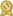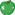Found 3800 calculus tutors – page 9 of 380.

Herndon 20171 · \$60/hour · teaches Algebra 2 - Calculus - Chemistry - Geometry - Praxis

I have taught Algebra I, Geometry, Algebra II, Trigonometry, Pre-Calculus, Math Analysis, Calculus AB, Calculus BC, and chemistry. My experience includes large classes, small groups, individual lessons...certified

Boulder 80302 · \$40/hour · teaches Bridge - Calculus - Chemistry - Chess - Cooking -

With multiple courses in high-level math and chemistry, I've had to learn everything from Differential Equations and Multi-variable Calculus to Quantum Mechanics and Thermodynamics. Specifically, I am proficient...certified

Agoura Hills 91301 · \$70/hour · teaches Pre-Calculus, Elementary, Elementary Math

Hello, my name is Mario. I tutor Math and I love what I do :) No payment, unless you are fully satisfied. This means that you don't have to pay unless you see the results - understand better and are getting...Green Apple award, member for 2 years and 7 months

Exeter 03833 · \$20/hour · teaches Calculus, Geometry, Math, Physics, Pre-Algebra

I scored an 800 on both the Math II and Physics SAT subject tests and a 5 on the AP Physics 1, AP Chemistry, and AP Calculus AB exams. I have experience tutoring in Math, Physics, and Chemistry, and I'm also capable...Green Apple award, member for 2 years and 7 months

New York 10001 · \$50/hour · teaches Calculus, Calligraphy, Career Planning, Cell

Preferred Subjects [math subjects (undergraduate level courses and select graduate level courses)] -- statistics, probability, calculus, differential equations, ODE, PDE, linear algebra, applied math...Green Apple award, certified, member for 4 years and 10 months

Laguna Hills 92653 · \$120/hour · teaches Calculus, Chinese, Differential Equations

I am one of only a few elite tutors in the country who have achieved a perfect score on the SAT (2400) and the ACT (36). My primary career is as an educational consultant. I devote over fifty hours a week...Green Apple award, certified, member for 4 years and 9 months

Lewes 19958 · \$25/hour · teaches ASVAB - Calculus - Common Core - Differential

...Pre-Calculus, Calculus and Math for Elementary Education Teachers. During my seven years teaching high school I taughcertified

Portland 06480 · \$40/hour · teaches Algebra 2 - Calculus - Geometry - Precalculus - SAT

I am currently teaching a Geometry course for students who are academically challenged, a Pre Calculus course , and AP Calculuscertified

Los Osos 93402 · \$40/hour · teaches Algebra 2 - Calculus - Geometry - Prealgebra -

I have taught all of Cuesta's math classes from arithmetic (Math 003 at Cuesta) through pre-calculus (Math 242) for the last 25 years. I also was the Math Lab coordinator for 12 years (until the program was...certified

Milford 03055 · \$40/hour · teaches Algebra 2 - Calculus - Differential Equations -

I have had many recent tutoring situations with students taking algebra, calculus, and statistics. Courses I have taught include algebra, trigonometry, precalculus, calculus, differential equations, statistics...certified